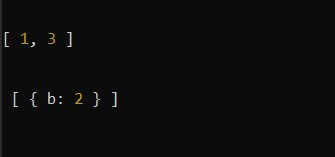# Lodash | _.differenceWith() Method

The _.differenceWith() method is similar to _.difference() method that returns the array containing the values that are in the first array not in the second array but in _.differenceWith() all the elements of the first array are compared with the second array by applying comparison provided in third. It may be a little complex to understand by reading this but it will become simple when you see the example.

Syntax:

`_.differenceWith(array, [values], [comparator])`

Parameters: This method accept three parameters as mentioned above and described below:

• array: This parameter holds the array that values are checked or inspect.
• values: This parameter holds the value that need to be removed.
• comparator: This parameter holds the comparison invoked per element.

Return Value: This method returns an array according to the condition explained above.

Example 1:

 `const _ = require(``'lodash'``) ` ` `  `let x = [1, 2, 3] ` ` `  `let y = [2, 4, 5] ` ` `  `let result = _.differenceWith(x, y, _.isEqual); ` ` `  `console.log(result); `

Here, `const _ = require('lodash')` is used to import the lodash library into the file.

Output:

`[1, 3]`

So, here each element of the first array is compared with each element of the second array according to the third comparator, in our case its _.isEqual. So, if the value becomes equal it removes it.

Example 2:

 `const _ = require(``'lodash'``); ` ` `  `let x = [{a: 1}, {b: 2}, 6] ` ` `  `let y = [{a: 1}, 7, 6] ` ` `  `let result = _.differenceWith(x, y, _.isEqual); ` ` `  `console.log(result); `

Output:

```[{b: 2}]
```

Example 3:

 `const _ = require(``'lodash'``); ` ` `  `let x1 = [1, 2, 3] ` ` `  `let y1 = [2, 4, 5] ` ` `  `let result1 = _.differenceWith(x1, y1, _.isEqual); ` ` `  `console.log(result1); ` ` `  `let x2 = [{a: 1}, {b: 2}, 6] ` ` `  `let y2 = [{a: 1}, 7, 6] ` ` `  `let result2 = _.differenceWith(x2, y2, _.isEqual); ` ` `  `console.log(``'\n\n'``, result2); `

Output:Note: This will not work in normal JavaScript because it requires the library lodash to be installed.My Personal Notes arrow_drop_upCheck out this Author's contributed articles.

If you like GeeksforGeeks and would like to contribute, you can also write an article using contribute.geeksforgeeks.org or mail your article to contribute@geeksforgeeks.org. See your article appearing on the GeeksforGeeks main page and help other Geeks.

Please Improve this article if you find anything incorrect by clicking on the "Improve Article" button below.

Article Tags :

Be the First to upvote.

Please write to us at contribute@geeksforgeeks.org to report any issue with the above content.